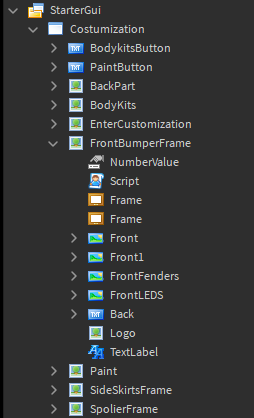# Increasing NumberValue

How can I increase NumberValue? I mean let’s say that Value is 0 and when I press E becomes 1 and when I press again it is made 2 and when I press Q returns to 1 and so on.

P.S.Is there a way to set a limit?

You can use `UserInputService.InputBegan` to make a NumberValue change value everytime you press a key. The thing that depends is if you want it local (only the client who pressed the key will see that number value change) or server sided (the server/everyone will notice that the value changed) and where the NumberValue is located

Not sure what you mean by this

I want it local and it is stored in a guiThe whole thing is that I want to use NumberValue as depending on the number, the CFrame from the camera to change

If you want the value to replicate to the server, you’ll need to use `RemoteEvent:FireServer()`.
Note: Do NOT pass the value with the FireServer function as any exploiter can change that value.

And yes: You can set a limit by doing ` Value = math.clamp(Value, MinValue, MaxValue)`

If you want it local, I.E, server cannot see the changes, you could do something like this in a LocalScript located anywhere it can be ran, probably StarterPlayerScripts would be a good choice

``````local uis = game:GetService("UserInputService")

local player = game:GetService("Players").LocalPlayer
local plrgui = player:WaitForChild("PlayerGui")

local screenGui = plrgui:WaitForChild("Costumization")
local bumperFrame = screenGui:WaitForChild("FrontBumperFrame")
local numVal = bumperFrame:WaitForChild("NumberValue")

local min = 0
local max = 20

uis.InputBegan:Connect(function(input,gpe)
if gpe then return end
local add = input.KeyCode == Enum.KeyCode.E and 1 or -1
numVal.Value = math.clamp(numVal.Value + add, min, max)
end)
``````
3 Likes

Here is some of what you’ll need to do
NumberValue will be named Numbers

So idk where you want to put it but make a GUI and a TextLabel, add the Numbers in the TextLabel and add a local script inside of it.

Setting the Numbers Limit
So let’s set the Number Value limit First.

``````Local Numbers = script.Parent.Value
—Default will be the default number you want to start off with. Min is the minimum and max is the maximum.
Numbers.math.clamp(Default, minimum, max)
``````

UserInputService
Now I hope I don’t make any mistakes in the following lines, I’ll correct myself if so:

``````local userInputService = game:GetService(“UserInputService”)